# 第 1 章 零基础入手 R

## 1.1 统计语言的历史

R语言是受Scheme语言（一种函数式编程语言）启发，并对S语言加以开源实现， 最新版本的R语言与早 期的S有一些重要的区别。R语言书由新西兰奥克兰大学的Ross Ihaka和Robert Gentleman创建，并由R Development Core团队开发，S语言之父Chambers也是R核心开发团队的成员。读者可能注意到，R语言 的命名以前两个R作者的名字首字母命名。R语言项目于1992年构思，1995年发布了最初版本，2000年2 月29日发布了稳定的beta版本（v1.0），截止2020年，已经到v4.0版本。

R的许多功能通过用户创建的R包得到扩展，这些包涵盖专门的统计技术，图形设备，导入/导出功能， 报告工具（Rmarkdown，knitr，Sweave）等。R的安装除了包含一组核心软件包，还有大量的发布到 CRAN， Bioconductor，Omegahat，GitHub等超过15,000个贡献软件包供使用。

## 1.2 R 语言介绍

“… a freely available language and environment for statistical computing and graphics which provides a wide variety of statistical and graphical techniques: linear and nonlinear modelling, statistical tests, time series analysis, classification, clustering, etc….”

#### R 工作目录

getwd()
#>  "/Users/ykang/Documents/Statistical-Computing-Chinese"

#### 安装 R 包

ggplot2 这个包为例，可以通过以下方式来安装。

• 先运行 RStudio，在“包”选项，点击“安装包”，并且安装 ggplot2 包。（请勾选“安装依赖包”选项）。

• 通过命令 install.packages('ggplot2')

#### 本书中的 R 示例

f <- expression(x^2 - 5)
df <- deriv(f, 'x', func = TRUE)

## 1.3 R 中的数据结构

R 是基于对象（objects）的，对象可以是 R 中的任何存在，如常数、数据结构、函数、图等。给定任何一个对象，可以通过 str() 来了解该对象的结构。R 中常用的基础数据结构有向量（vector）、列表（list）、矩阵（matrix）、数据框（data frame）等。

### 1.3.1 向量

num_x <- c(1.2, 4.5, 6)
int_x <- c(1L, 6L, 80L)
log_x <- c(TRUE, FALSE, T, F, F)
cha_x <- c('yanfei', 'feng')
cha_x
#>  "yanfei" "feng"

10:15
#>  10 11 12 13 14 15
seq(from=1, to=10, by=3)
#>   1  4  7 10
LETTERS[1:10]
#>   "A" "B" "C" "D" "E" "F" "G" "H" "I" "J"
rep(14, 10)
#>   14 14 14 14 14 14 14 14 14 14

### 1.3.2 列表

l <- list(a = c(1, 2), b = "apple", c = list(1,2))
l
#> $a #>  1 2 #> #>$b
#>  "apple"
#>
#> $c #>$c[]
#>  1
#>
#> $c[] #>  2 str(l) #> List of 3 #>$ a: num [1:2] 1 2
#>  $b: chr "apple" #>$ c:List of 2
#>   ..$: num 1 #> ..$ : num 2

### 1.3.3 矩阵

m <- matrix(c(1:6), 2, 3)
m
#>      [,1] [,2] [,3]
#> [1,]    1    3    5
#> [2,]    2    4    6

dim() 函数可以得到矩阵的维度，t() 可以得到矩阵的转置：

dim(m)
#>  2 3
t(m)
#>      [,1] [,2]
#> [1,]    1    2
#> [2,]    3    4
#> [3,]    5    6

rbind()cbind() 可以将两个矩阵按行或者按列合并：

n <- matrix(c(8:13), 2, 3)
rbind(m, n)
#>      [,1] [,2] [,3]
#> [1,]    1    3    5
#> [2,]    2    4    6
#> [3,]    8   10   12
#> [4,]    9   11   13
cbind(m, n)
#>      [,1] [,2] [,3] [,4] [,5] [,6]
#> [1,]    1    3    5    8   10   12
#> [2,]    2    4    6    9   11   13

### 1.3.4 数据框

df <- data.frame(x = 1:10, y = letters[1:10])
str(df)
#> 'data.frame':	10 obs. of  2 variables:
#>  $x: int 1 2 3 4 5 6 7 8 9 10 #>$ y: Factor w/ 10 levels "a","b","c","d",..: 1 2 3 4 5 6 7 8 9 10

df <- data.frame(x = 1:3)
df$y <- list(1:2, 1:3, 1:4) df #> x y #> 1 1 1, 2 #> 2 2 1, 2, 3 #> 3 3 1, 2, 3, 4 ### 1.3.5 子集提取 在 R 中有三种操作符可以提取不同数据结构的子集： 1. [ 可以返回与原数据相同的数据类型。 2. [[ 用于提取列表和数据框的子集。 3. $ 可以依据变量名字提取列表和数据框的子集。

#### 提取向量的子集

x <- 1:10
x  # 提取第一个元素
#>  1
x  # 提取第二个元素
#>  2
x[c(1, 3, 4)] # 提取第1、3、4个元素
#>  1 3 4
x[x > 2] # 提取大于2的元素
#>   3  4  5  6  7  8  9 10

#### 提取列表的子集

x <- list(a = 1:4, b = letters[1:4], d = c(TRUE, FALSE))
x[] # 提取x的第一个元素
#>  1 2 3 4
x[['b']] # 提取x的b元素
#>  "a" "b" "c" "d"
x$a # 提取x的a元素 #>  1 2 3 4 x # [] 返回的数据结构和x相同，还是一个列表 #>$a
#>  1 2 3 4
x[c(1,3)]
#> $a #>  1 2 3 4 #> #>$d
#>   TRUE FALSE

#### 提取矩阵的子集

x <- matrix(1:6, 2, 3)
x[1, 2] # 提取x的第一行第二列
#>  3
x[, 2] # 提取x的第二列
#>  3 4

#### 提取数据框的子集

x <- data.frame(a = 1:10, b = letters[1:10], d = rnorm(10))
#>  3
#>
#>  3
#>
#> $b #>  -0.2262 sapply(x, mean) #> a b #> 3.0000 -0.2262 x <- 1:4 lapply(x, runif) #> [] #>  0.7996 #> #> [] #>  0.6798 0.9041 #> #> [] #>  0.1540 0.4775 0.2319 #> #> [] #>  0.4398 0.8341 0.4688 0.9288 sapply(x, runif) #> [] #>  0.1738 #> #> [] #>  0.3077 0.8567 #> #> [] #>  0.8124 0.3650 0.1968 #> #> [] #>  0.1180 0.8431 0.5125 0.5365 我们看到在第一个例子中 sapply() 输出的是一个向量，简化了 lapply() 的输出。但是在第二个例子中，sapply() 输出的仍然是一个列表，因为在 lapply() 输出的列表中每个元素的长度不同。假若长度相同，sapply() 则可以简化结果如下： x <- rep(3, 4) lapply(x, runif) #> [] #>  0.6775 0.9967 0.3191 #> #> [] #>  0.9657 0.0935 0.3567 #> #> [] #>  0.5989 0.2656 0.7416 #> #> [] #>  0.2106 0.1453 0.4369 sapply(x, runif) #> [,1] [,2] [,3] [,4] #> [1,] 0.5173 0.5190 0.4298 0.13778 #> [2,] 0.3215 0.9658 0.4672 0.61509 #> [3,] 0.5834 0.4502 0.1323 0.05738 #### apply() apply() 可以对一个矩阵（或多维数组）的行或者列执行一个函数，如： options(width = 50) x <- matrix(rnorm(200), 10, 20) # 对矩阵 x 的列求均值 apply(x, 2, mean) #>  0.17437 0.07463 -0.31853 0.10154 -0.12579 #>  -0.08245 0.52580 0.00473 0.16940 -0.27254 #>  0.12730 0.18771 -0.11926 -0.14678 -0.33212 #>  -0.21819 -0.70370 -0.13478 0.23316 -0.40439 # 对矩阵 x 的每一行求 20% 和 75% 分位数 apply(x, 1, quantile, probs = c(0.25, 0.75)) #> [,1] [,2] [,3] [,4] [,5] #> 25% -0.2880 -0.8593 -0.8059 -0.8780 -0.86641 #> 75% 0.8355 0.3652 0.5570 0.4038 0.06178 #> [,6] [,7] [,8] [,9] [,10] #> 25% -0.6624 -0.8198 -1.267 -0.86620 -0.1495 #> 75% 0.8106 1.0479 1.215 0.07795 0.9440 #### tapply() tapply() 可以对一个向量的子集执行一个函数。比如在下述例子中我们分别得到来自不同分布随机数的均值。 x <- c(rnorm(100), runif(100), rt(100, 2)) # 定义一个因子型的分组变量 f <- gl(3, 100) # 求每一组的均值 tapply(x, f, mean) #> 1 2 3 #> 0.1966 0.4892 0.3170 #### mapply() mapply() 适用于多元情形。比如: mapply(rep, 1:4, 4:1) #> [] #>  1 1 1 1 #> #> [] #>  2 2 2 #> #> [] #>  3 3 #> #> [] #>  4 可以代替繁琐的重复编程： list(rep(1, 4), rep(2, 3), rep(3, 2), rep(4, 1)) #> [] #>  1 1 1 1 #> #> [] #>  2 2 2 #> #> [] #>  3 3 #> #> [] #>  4 mapply() 在当需要将函数向量化时非常有用，比如： f <- function(x = 1:3, y) c(x, y) f(1:3, 1:3) #>  1 2 3 1 2 3 mapply(f, 1:3, 1:3) #> [,1] [,2] [,3] #> [1,] 1 2 3 #> [2,] 1 2 3 对于函数 f()，我们对比 f(1:3, 1:3)mapply(f, 1:3, 1:3) 可以很容易的理解 mapply() 可以将两对向量的元素分别传递给 f()。R 函数 Vectorize() 也可以通过向量化自动实现相同的效果： vf <- Vectorize(f) vf(1:3,1:3) #> [,1] [,2] [,3] #> [1,] 1 2 3 #> [2,] 1 2 3 ## 1.6 如何写 R 函数 什么时候应该写一个 R 函数呢？当你发现你在重复执行一段代码（可能只对其中的参数做了细微改变）时，这时候就应该考虑写 R 函数了！在 R 中的函数是通过 function() 来定义的，它向其它的 R 对象一样，是以对象的形式存在的： name <- function(arg_1, arg_2, …) { expression return(value) } 其中 expression 是一段 R 代码，通过参数 arg_1, arg_2, … 来计算函数的输出 value。函数的调用可以用 name(expr_1, expr_2, …)。下面我们来看一个例子： f <- function(num = 1) { myname <- "Yanfei \n" for (i in seq_len(num)) { cat(myname) } myname.length <- nchar(myname) * num return(myname.length) } mynamel <- f(3) #> Yanfei #> Yanfei #> Yanfei print(mynamel) #>  24 在这个例子中，我们定义了函数 f()，其中 1. f() 有一个参数 num，这个函数中设置了默认值 num = 1，因此如果我们执行 f()，则默认参数 num = 1。如果不设置默认值，执行 f() 就会报错。 2. f() 循环打印 “Yanfei”，打印次数是由参数 num 设定的。 3. 函数的输出为打印到控制台中的字符个数。 ## 1.7 如何在 R 中绘图 “The simple graph has brought more information to the data analyst’s mind than any other device.” – John Tukey 在 R 中绘图的方式有很多种，如 R 中的基础绘图（base graphics，基于 graphics 包）、基于 ggplot2(Wickham 2016)、基于 lattice(Sarkar 2008)、基于 plotly(Sievert 2020) 等等。 ### 1.7.1 R 中的基础绘图 R 中的基础做图包含用于绘制最常见图形的函数，如适用于定量数据的条形图、饼图，以及适用于定性数据的直方图、箱线图、折线图、散点图等。 此外，还可以绘制分位数（QQ）图，等高线图、三维图等。 graphics 包中包含的常用的绘图函数如表 1.1 所示 (Adler 2010) 表1.1: R 中的基础绘图函数 R 函数 描述 barplot() 条形图 pie() 饼图 hist() 直方图 density() 核密度图 boxplot() 箱线图 plot() 散点图 smoothScatter() 带有平滑密度的散点图 pairs() 两两散点图 image() 图像 contour() 等高线图 persp() 三维图 #### 条形图 条形图通常用于展示定性变量。以 2019 年中国居民人均消费支出（来源：国家统计局网站）为例： expense <- data.frame( 消费 = c(6084, 5055, 2862, 2513, 1902, 1338, 1281, 524), 类别 = c("食品烟酒", "居住", "交通通信", "教育文化娱乐", "医疗保健", "衣着", "生活用品及服务", "其他用品及服务")) par(family = 'SimHei') barplot(height = expense$消费,
main = "2019 年中国居民人均消费支出",
ylab = "消费（元）",
labels = expense类别, main = "2019 年中国居民人均消费支出结构")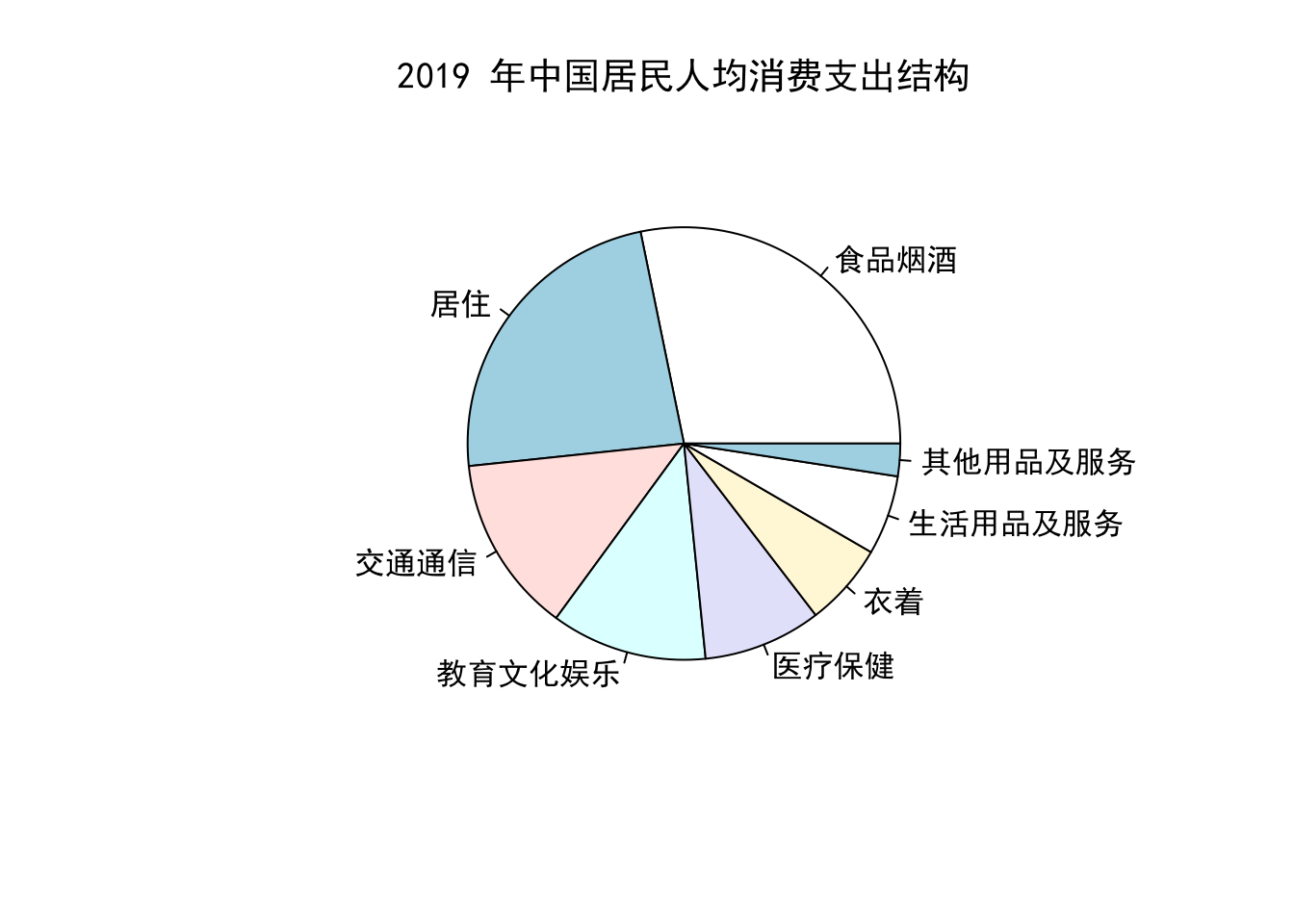#### 直方图 以下为某个班级 50 名同学的《统计计算》课程考试成绩。 #>  100 84 88 83 72 79 87 91 80 74 89 #>  83 91 94 95 96 74 88 96 94 97 94 #>  78 98 72 77 95 76 79 78 99 88 73 #>  83 86 80 76 90 81 84 79 82 76 78 #>  78 79 92 96 97 90 从这组数据中我们可以了解这个班级同学的《统计计算》成绩分布情况，比如我们可以统计出成绩属于每个区间段的学生个数（如表 1.2 所示）。 表1.2: 某班级《统计计算》成绩分布情况 成绩区间 学生数 (70, 75] 5 (75, 80] 14 (80, 85] 7 (85, 90] 8 (90, 95] 8 (95, 100] 8 直方图可以以图的形式展示以上分布。 par(family = 'SimHei') hist(grades, breaks = 4, col = 'orange3', border='darkblue', main = '某班级《统计计算》考试成绩直方图', xlab = '成绩', ylab = '频数')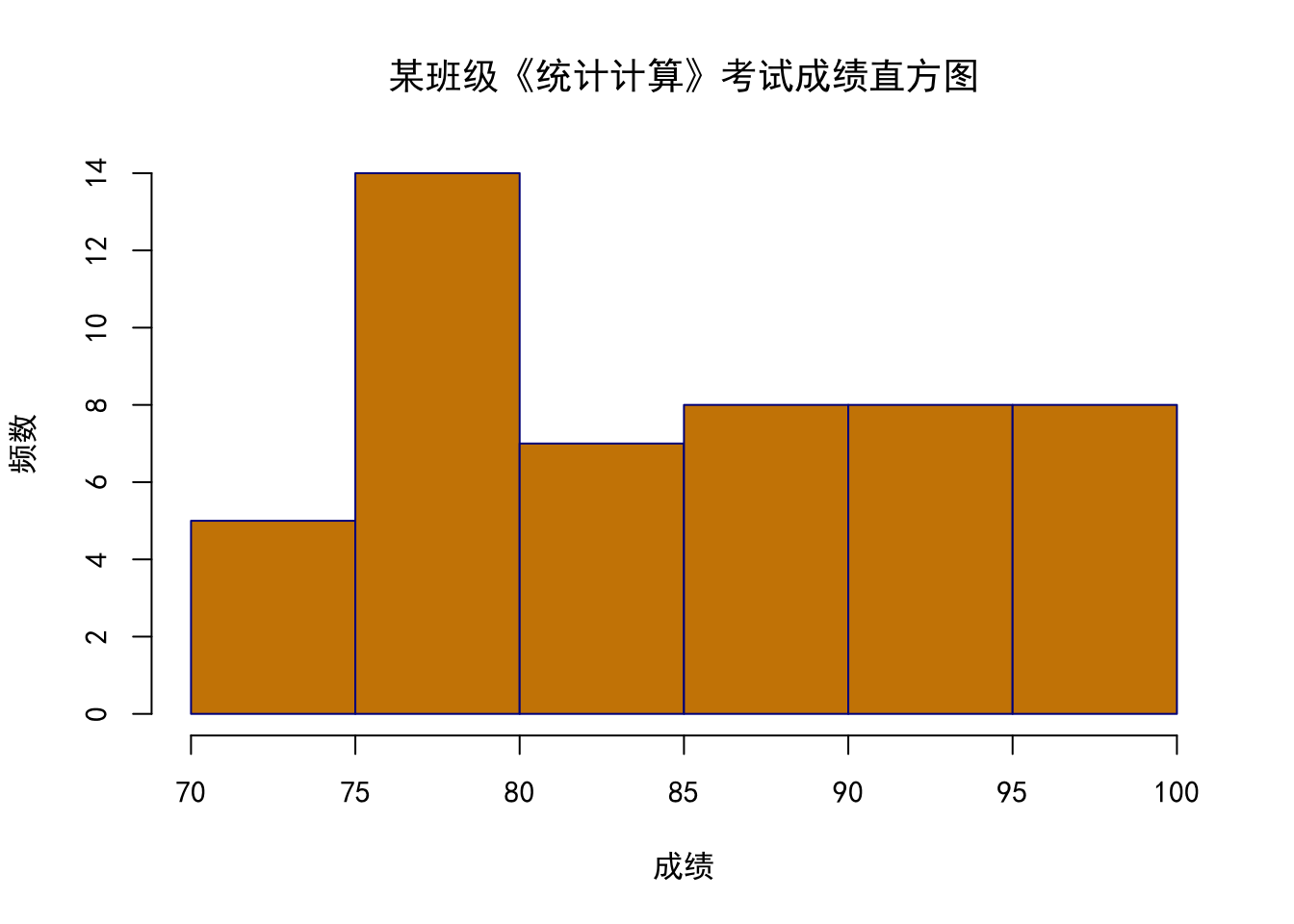#### 箱线图 箱线图可以基于一组数据的四分位数（quartiles）来展示数据分布。一个一般的箱线图可以展示： 1. “最小值”：去除异常值外的最小值。 2. “最大值”：去除异常值外的最大值。 3. 中位数：一组数据中位于中间位置的数，也叫第二四分位数（the second quartiile，简称 $$Q_2$$）或第50百分位数（50th percentile）。 4. 第一四分位数（the first quartiile，简称 $$Q_1$$），也叫第25百分位数（25th percentile）。 5. 第三四分位数（the third quartiile，简称 $$Q_3$$），也叫第75百分位数（75th percentile）。 其中“最小值”和“最大值”通过四分位距（Interquartile range，简称IQR = $$Q_3$$ - $$Q_1$$）来确定： \begin{aligned} “最小值” &= Q_1- 1.5*\mathrm{IQR}, \\ “最大值” & = Q3+ 1.5*\mathrm{IQR}. \end{aligned} 下面我们通过箱线图来展示《统计计算》成绩分布情况。 par(family = 'SimHei') boxplot(grades, col = 'orange3', main = '统计学考试成绩箱线图')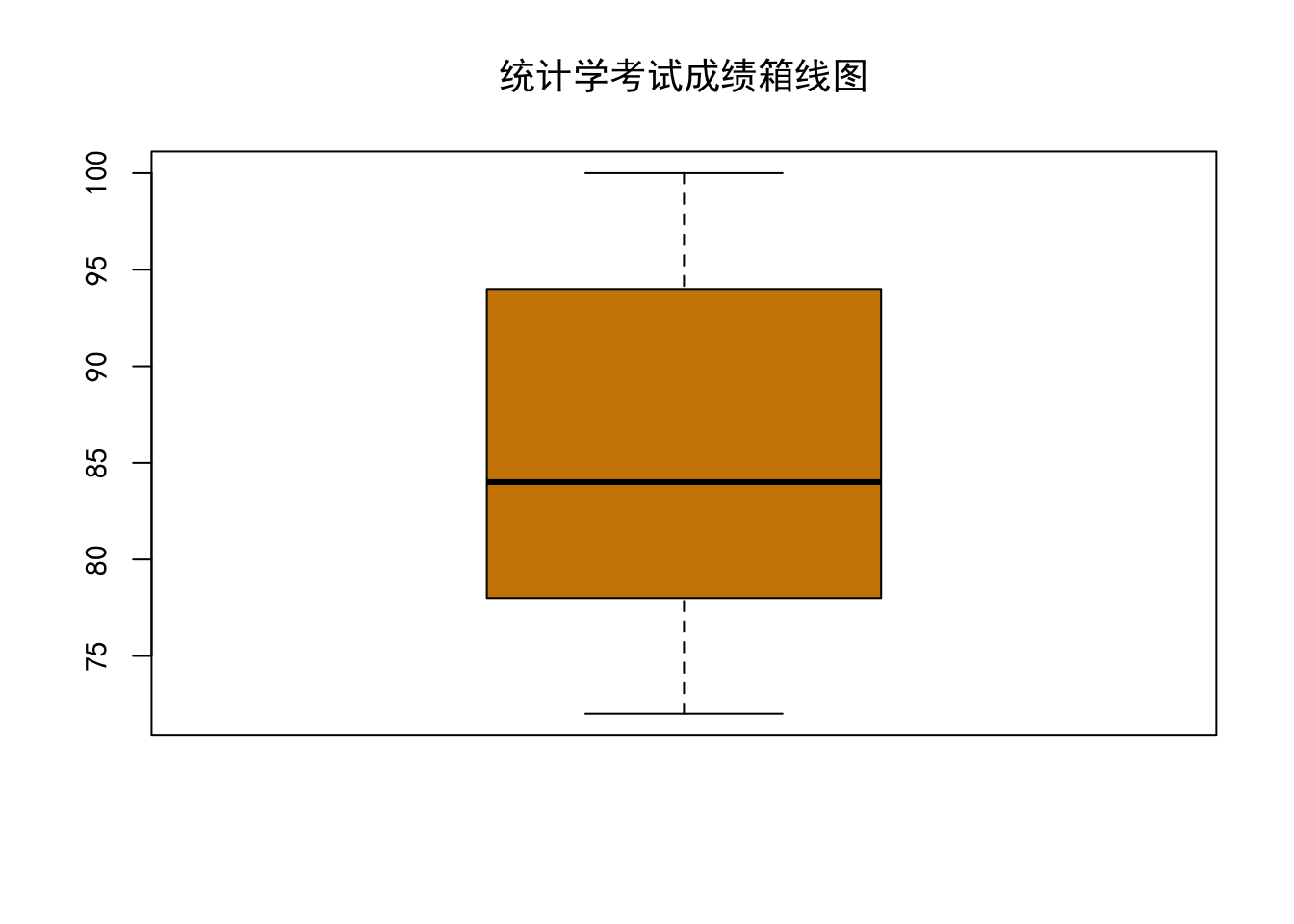#### 散点图 散点图可以反映两个变量之间的相关关系，例如下图展示了体重与腹围之间的关系。 library(faraway) data(fat) par(family='SimHei') plot(fatabdom, fat\$weight,
col="#69b3a2",
xlab="腹围", ylab="体重", pch = 16,
main="腹围和体重")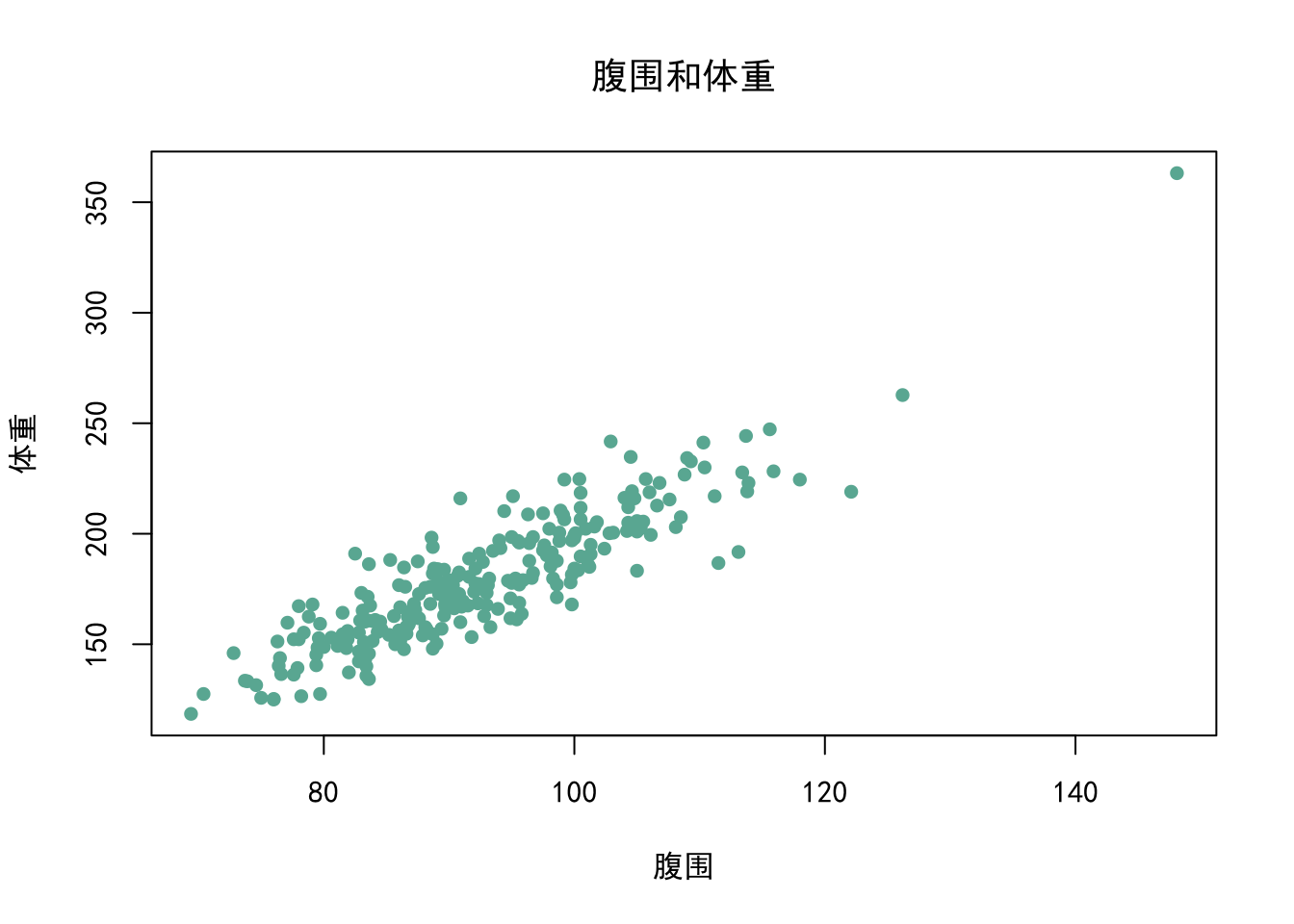### 1.7.2ggplot2

ggplot2是 R 中最美观的绘图框架之一，它有一个精心设计的绘图结构。ggplot2 绘图是基于图形语法（The Grammar of Graphics）的， 这导致它与基础绘图方式非常不同：

1. ggplot2 绘图所需的所有数据通常包含在数据框中，数据框可以通过 ggplot() 或者对应的 geoms 来提供（稍后会有更多细节）。
2. 您可以通过向使用 ggplot() 创建的现有绘图添加更多层（和主题）来不断增强绘图。

#### 第一步： 初始化

library(ggplot2)
ggplot(diamonds)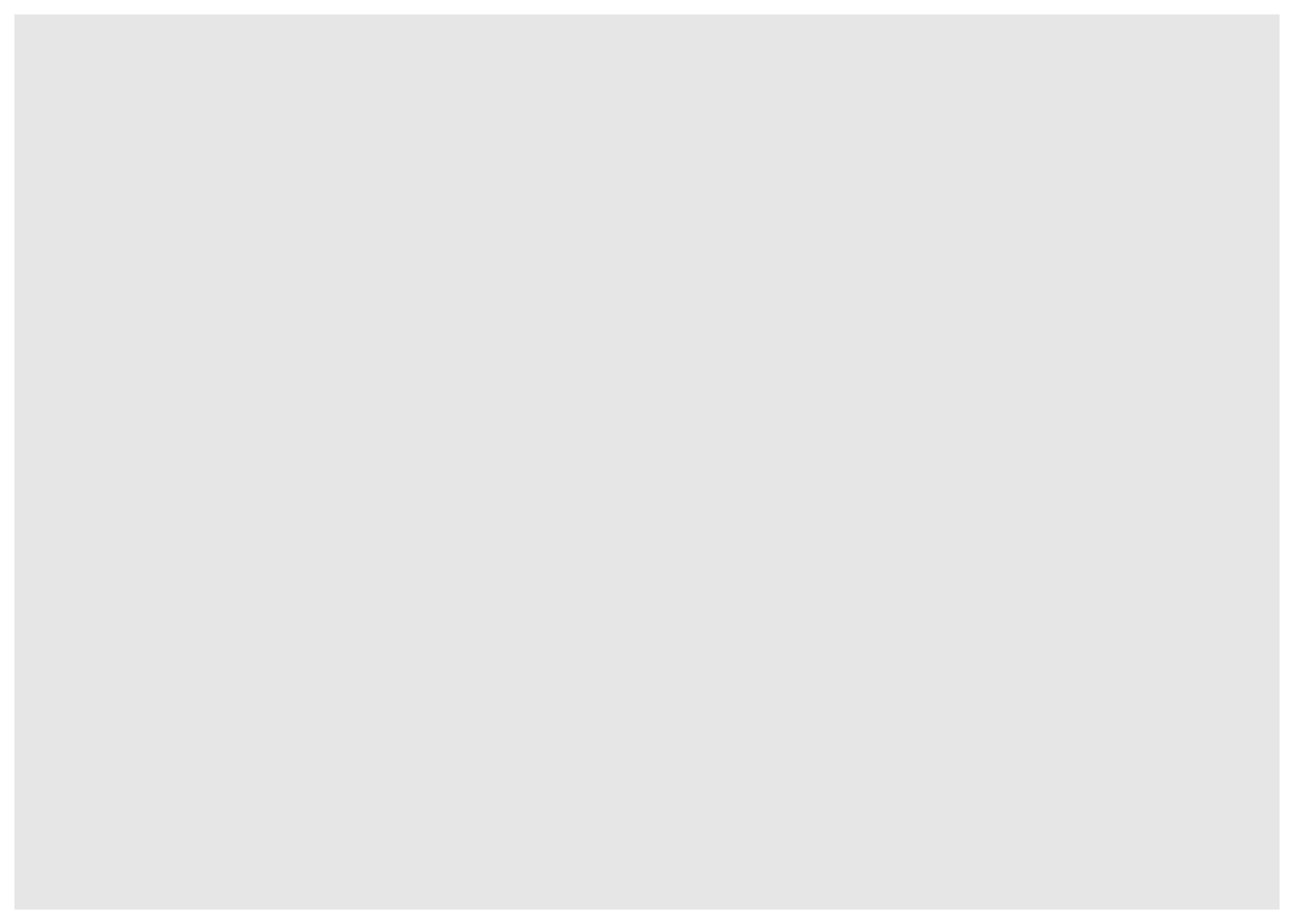ggplot(diamonds, aes(x = carat, y = price))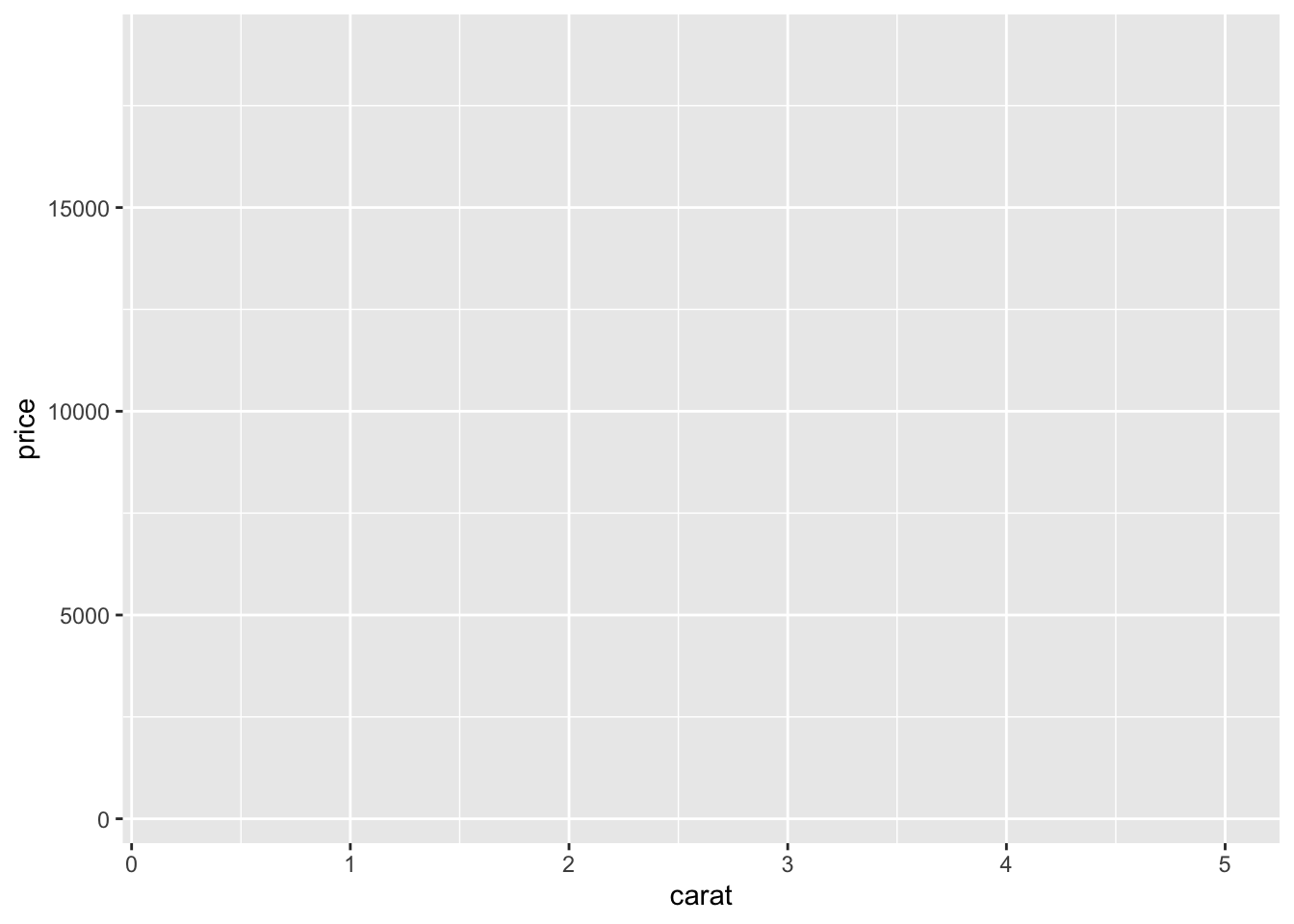ggplot(diamonds, aes(x = carat, color = cut))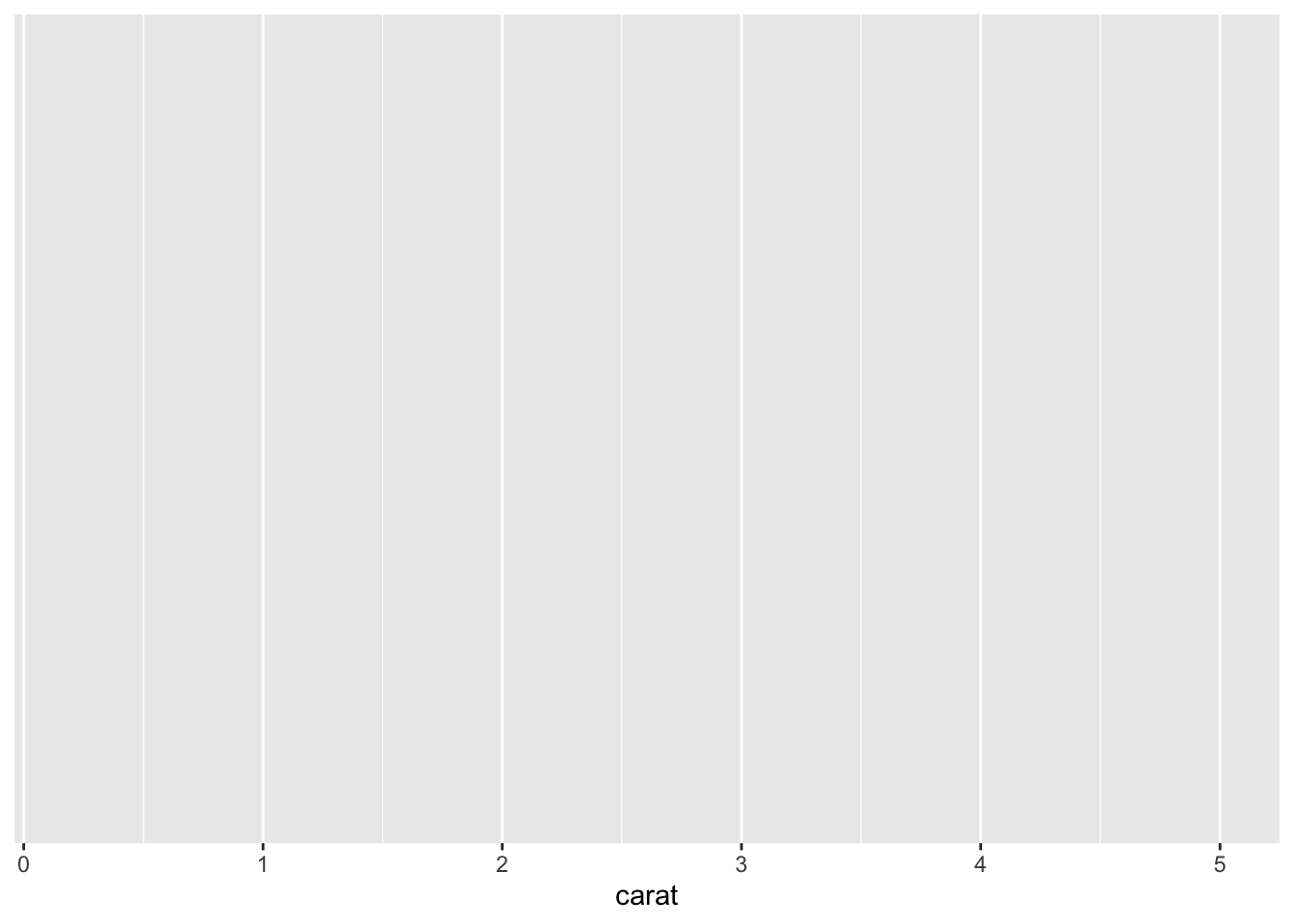在这种情况下，cut 变量的每一个类别会展现成不同的颜色。如果你想固定颜色或者大小，需要在 aes() 参数外设置，如：

ggplot(diamonds, aes(x = carat), color = 'orange3')#### 第二步： 加图层

ggplot2 中的图层（layers）也叫 “geoms” 。初始化之后，就可以一层一层的加图层了。比如我们可以通过 geom_point() 加入散点图层。

ggplot(diamonds, aes(x = carat, y = price)) +
geom_point()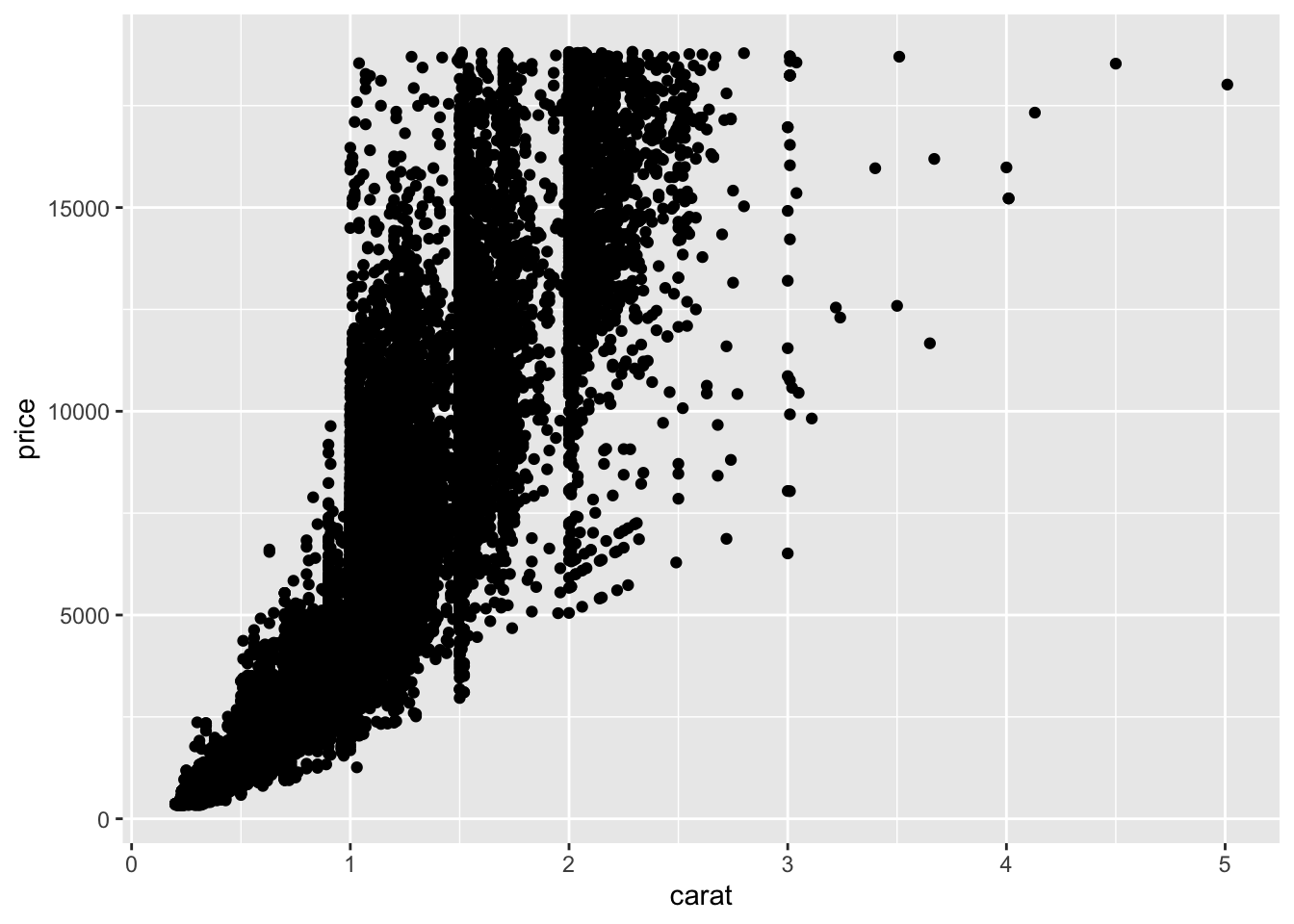aes() 也可以放在图层 geom_point() 里。

ggplot(diamonds) +
geom_point(aes(x = carat, y = price))ggplot(diamonds, aes(x = carat, y = price)) +
geom_point() +
geom_smooth()ggplot(diamonds, aes(x = carat, y = price, color = cut)) +
geom_point() +
geom_smooth()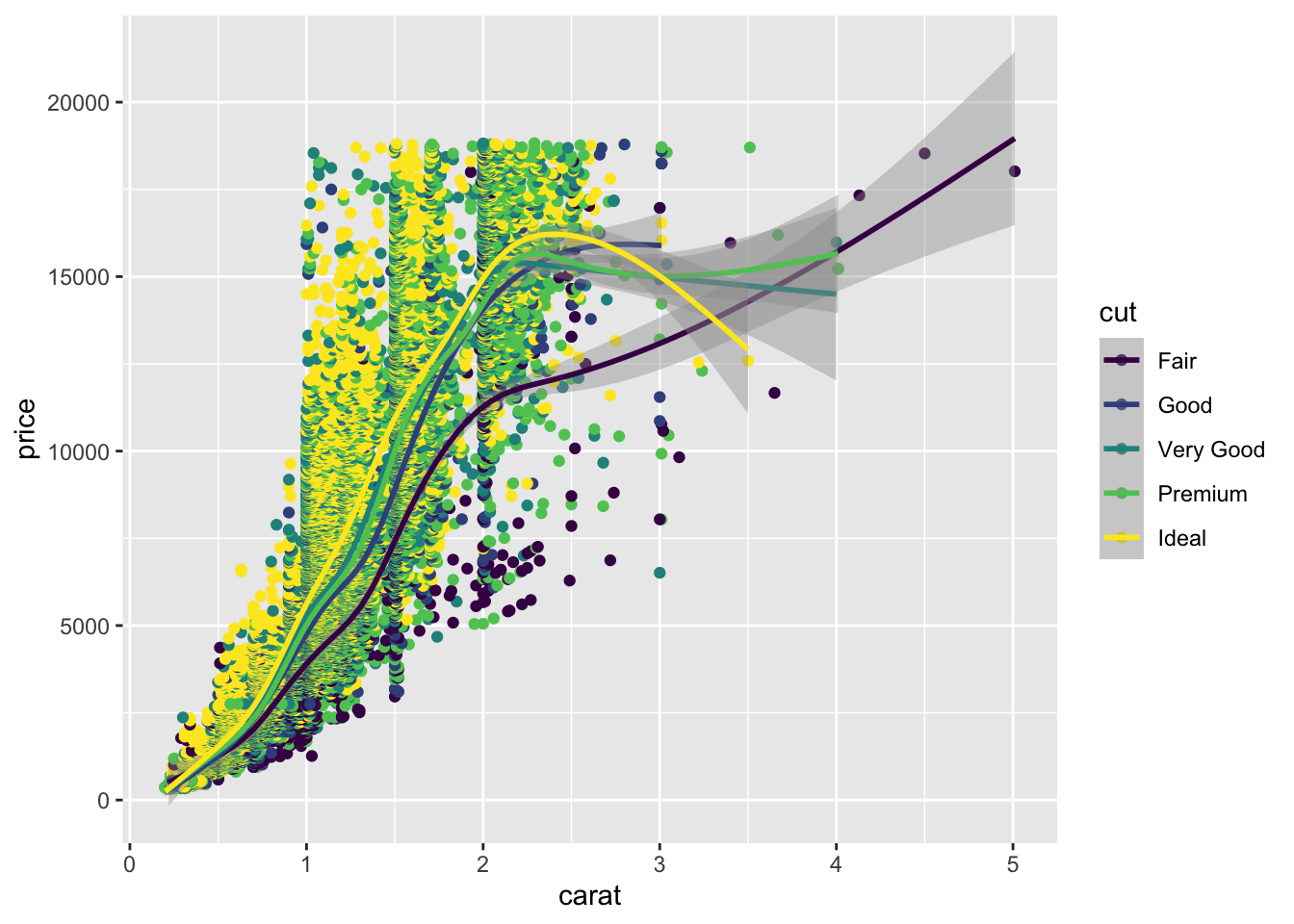ggplot(diamonds, aes(x = carat, y = price, color = cut, shape = color)) +
geom_point() +
geom_smooth()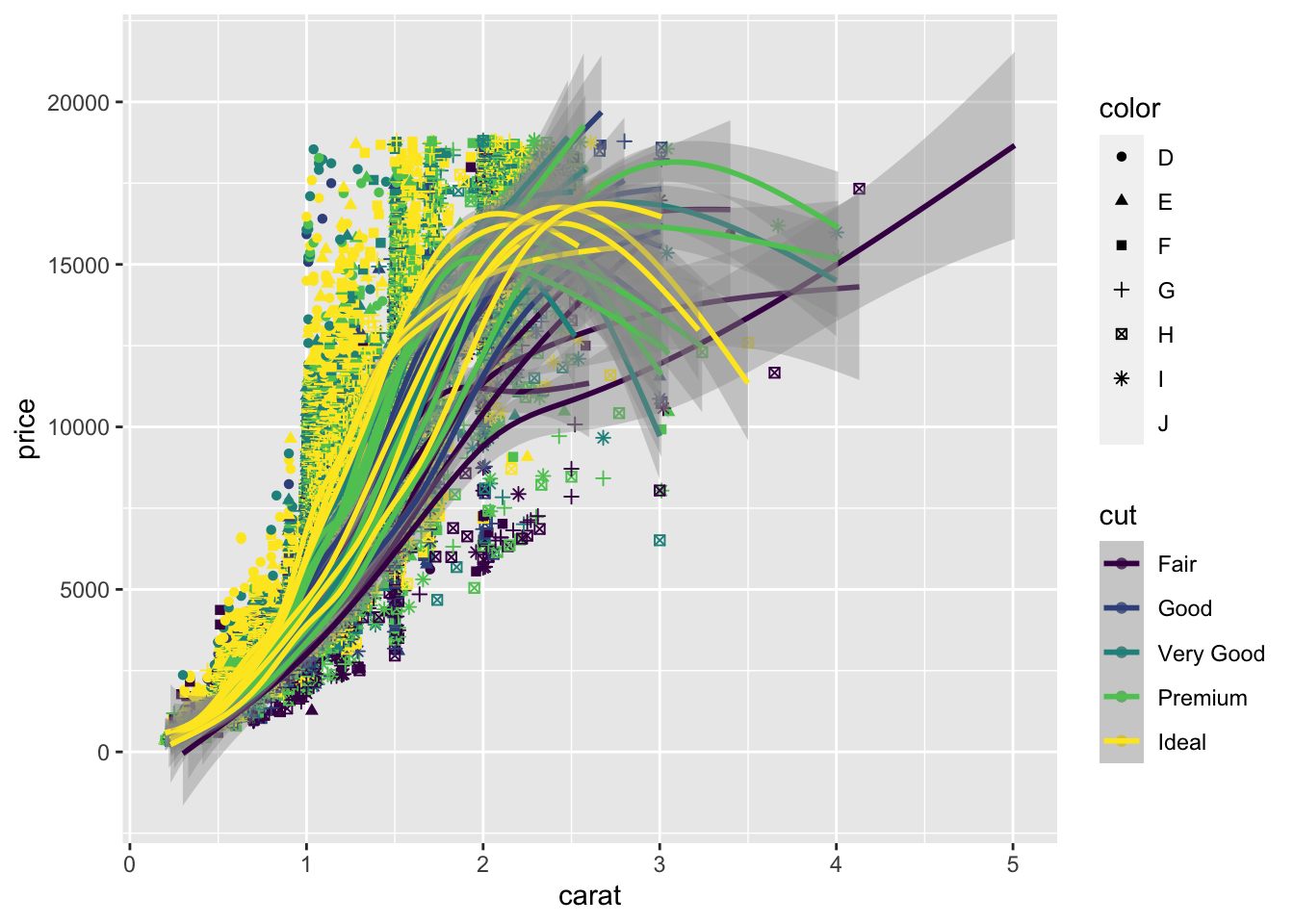#### 第三步： 加标签

ggplot(diamonds) +
geom_point(aes(x = carat, y = price, color = cut)) +
labs(title = 'Scatterplot of carat and price',
x = 'Carat', y = 'Price')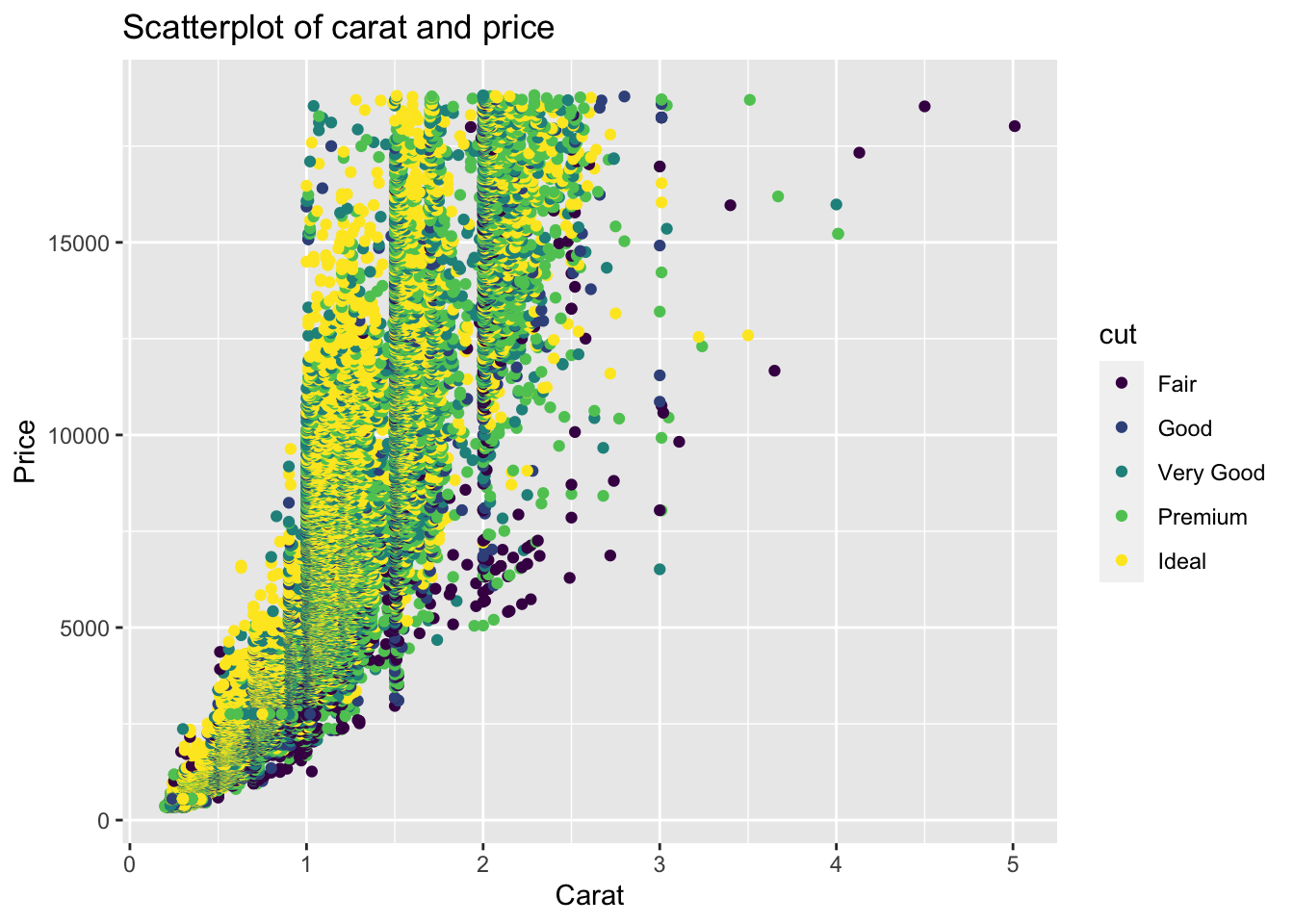#### 第四步： 修改主题

p <- ggplot(diamonds) +
geom_point(aes(x = carat, y = price, color = cut)) +
labs(title = 'Scatterplot of carat and price',
x = 'Carat', y = 'Price')
p1 <- p + theme(plot.title = element_text(size=20, face="bold"),
axis.text.x = element_text(size=15),
axis.text.y = element_text(size=15),
axis.title.x = element_text(size=20),
axis.title.y = element_text(size=20))
p1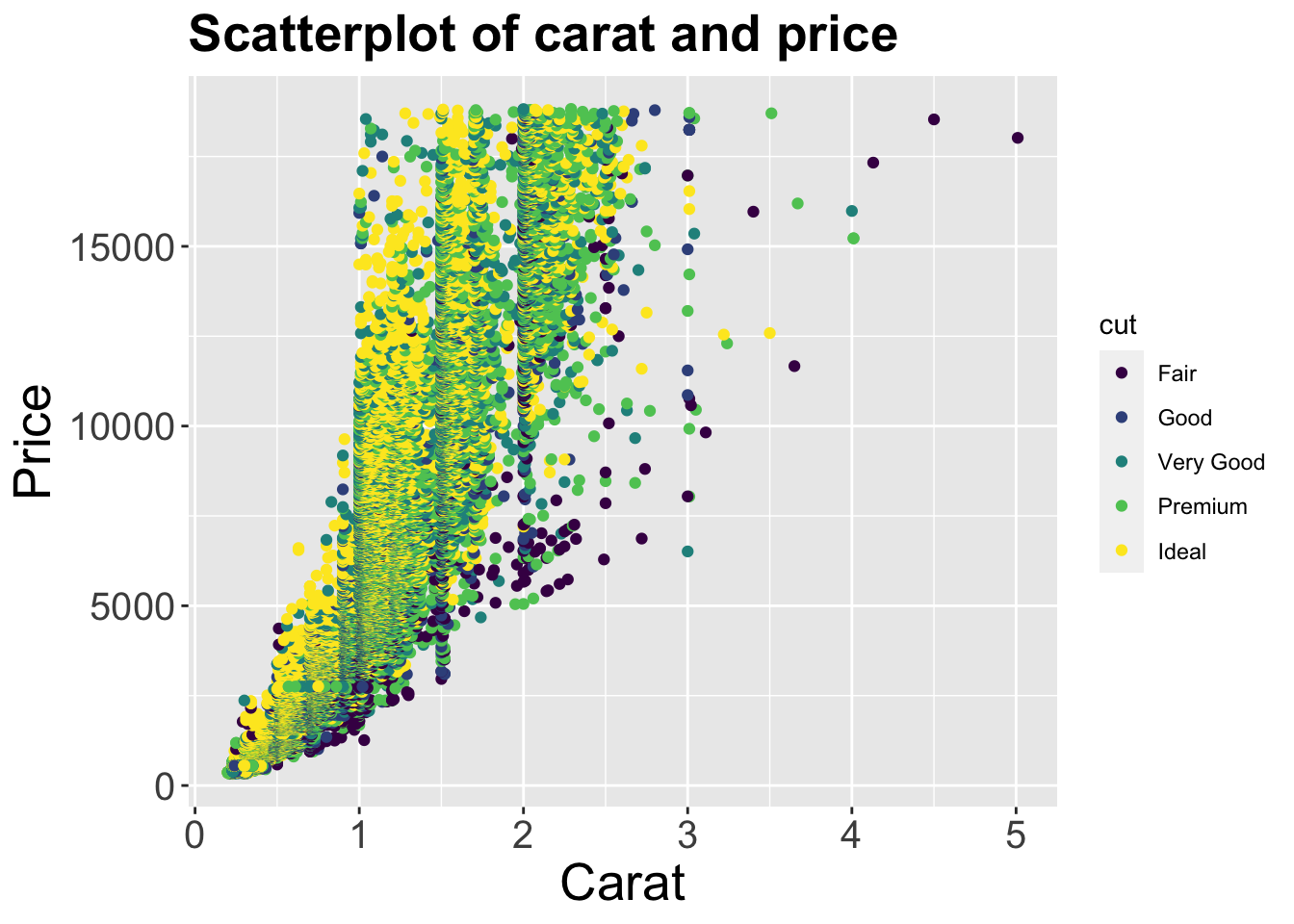p1 + scale_color_discrete(name = "Cut of diamonds")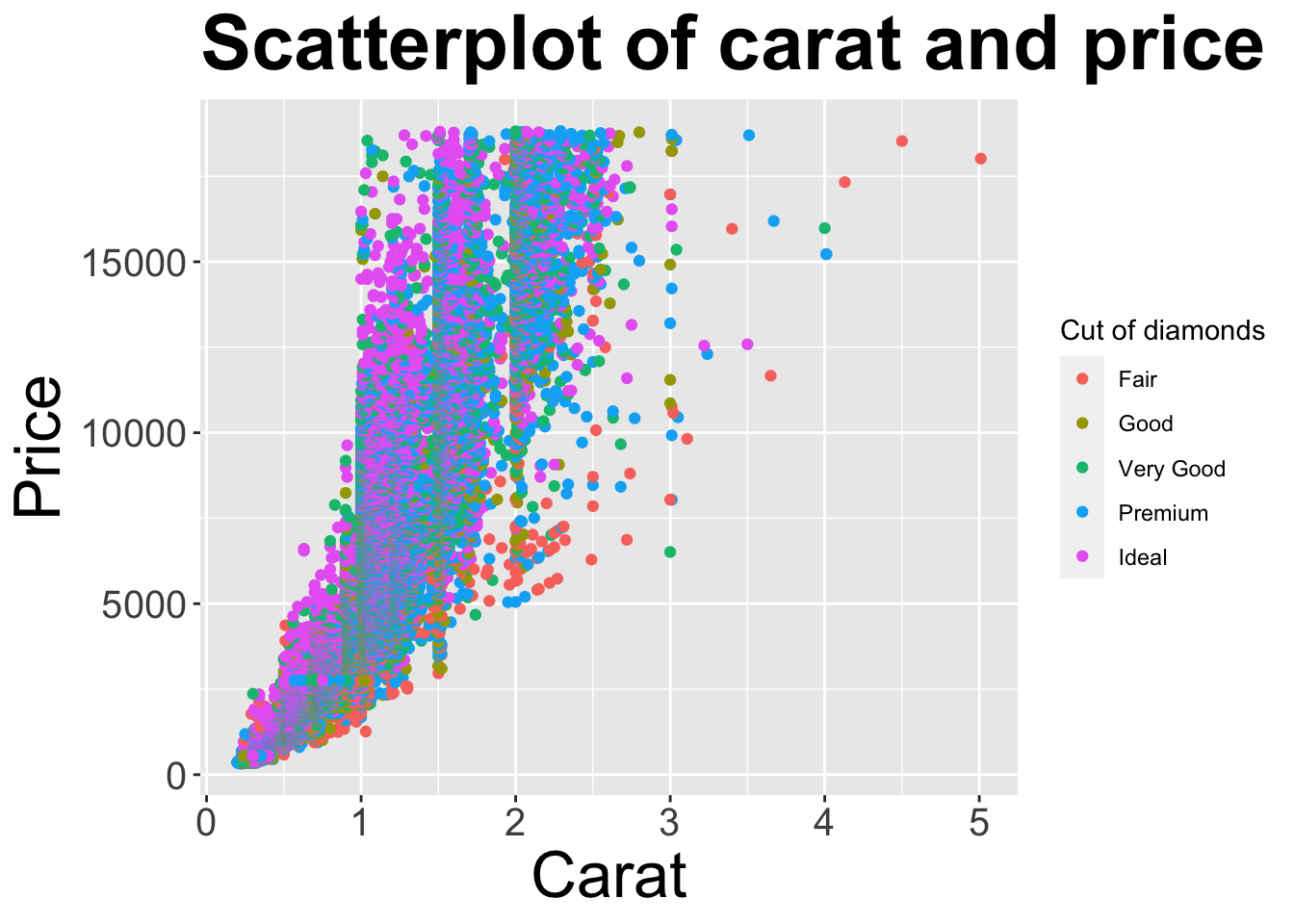如果图例所对应的属性是 shape，则可以使用 scale_shape_discrete() 来调整。

## 1.8 有关 R 的学习资源

### 参考文献

Adler, Joseph. 2010. R in a Nutshell: A Desktop Quick Reference. " O’Reilly Media, Inc.".

Sarkar, Deepayan. 2008. Lattice: Multivariate Data Visualization with R. New York: Springer. http://lmdvr.r-forge.r-project.org.

Sievert, Carson. 2020. Interactive Web-Based Data Visualization with R, Plotly, and Shiny. Chapman; Hall/CRC. https://plotly-r.com.

Wickham, Hadley. 2016. Ggplot2: Elegant Graphics for Data Analysis. Springer-Verlag New York. https://ggplot2.tidyverse.org.